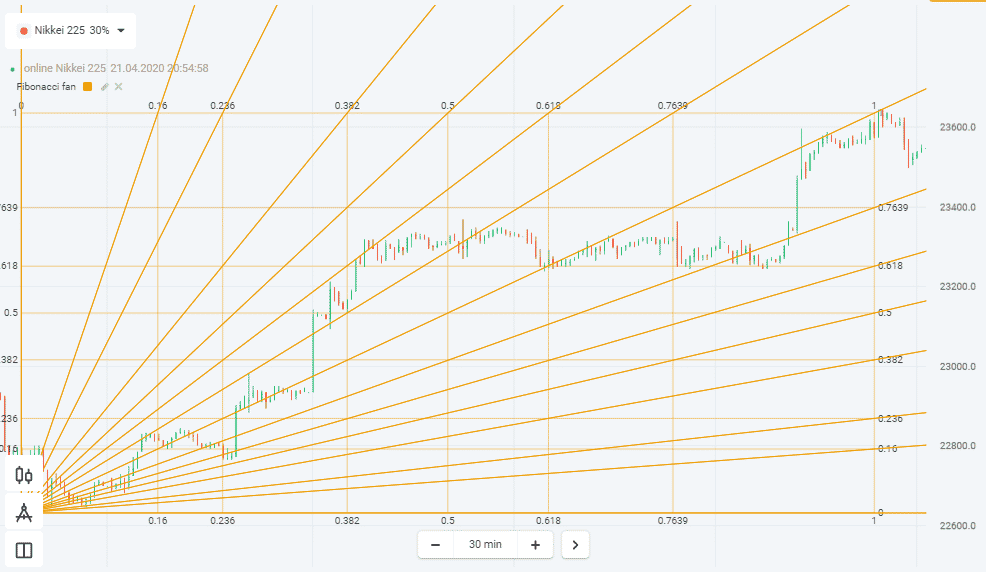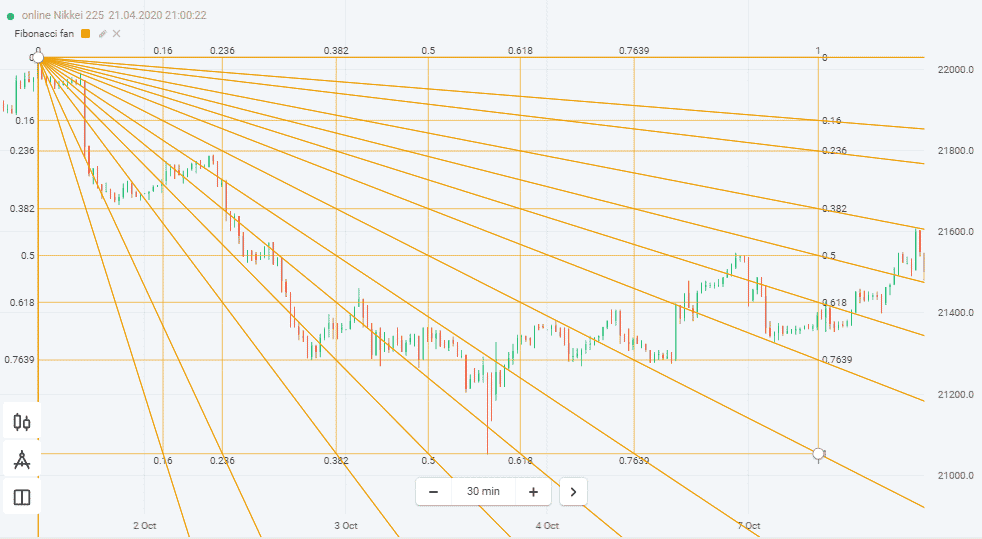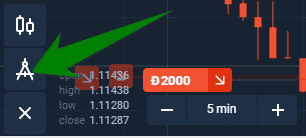# How to use the Fibonacci Fan Indicator to trade in Olymp Trade

Visit Website.
Features
What\'s this?
Rating
REGISTER
• Deposit \$10
• No withdrawal limit
• FREE demo account
Get FREE \$10,000 in Your Demo Account.

You already know about the Fibonacci sequence and of course, Fibonacci levels.

In a nutshell, these are mathematical calculations based on the golden ratio that you can apply to trade on Olymp Trade.

Fibonacci sequence is the series of numbers: 1, 2, 3, 5, 8, 13, 21, etc. Looking at it, you will realize that each next number is the sum of two preceding numbers.

### Other Posts Like This

Now, these series of numbers have found their way into online trading where traders leverage the Fibonacci sequence to generate support and resistance level.

While we will not be delving into all these today, you are in for a treat.

Why?

Visit Website.
Features
What\'s this?
Rating
REGISTER
• Deposit \$10
• No withdrawal limit
• FREE demo account
Get FREE \$10,000 in Your Demo Account.

Because there is more to the Fibonacci sequence than Fibonacci levels. And that’s just part of our discussion for today.

But even more interesting, I will walk you through issues as important as; how to trade using a Fibonacci fan on Olymp Trade; Fibonacci fan trading strategies and how to apply the indicator to your daily trading charts.

Let’s get right to it, shall we?

## What is Fibonacci Fan?

Fibonacci fan is an indicator on Olymp Trade that is specifically used for analyzing trends.

Visit Website.
Features
What\'s this?
Rating
REGISTER
• Deposit \$10
• No withdrawal limit
• FREE demo account
Get FREE \$10,000 in Your Demo Account.

Most traders know it as the Fibo fan.

Basically, the Fibonacci fan is plotted on the trading chart as lines showing resistance and support levels within a prevailing trend.

In other words, the Fibonacci fan leverages Fibonacci ratios to come up with potential support and resistant points on a trend.

## Understanding Fibonacci Fan in Olymp Trade.

You might be wondering why that name.

Well, as it turns out, the Fibonacci fan indicator when fully plotted on the trading chart actually looks like a fan.

In fact, the indicator is comprised of three trend lines running from either trough or peak of a trend through the points dictated by Fibonacci retracements.

These are 23.6%, 38.2%, 50%, and 61% retracement levels.

To draw it, all you have to do is pick a trend line as the base for the Fibonacci fan. Commonly, it is drawn in such a way that it covers the lowest price point all the way to the highest price point over a specific period of time.

Once you do that, it is time to come up with retracement levels.

And to do that, you have to divide price difference on both the low and highest level by the ratios derived from the Fibonacci sequence.

As we have already mentioned, the common ratios used are 23.6 percent, 38.2 percent, 50 percent, and 61.8 percent.

When you connect the trend line starting point to each of these retracement levels, you get the Fibonacci fan indicator on Olymp Trade.

Before we look at Fibonacci fan interpretations, let’s first understand how it calculated.

## How is the Fibonacci fan calculated and drawn?

Here is the thing:

Fibonacci sequence is based on complex mathematical computations and I would hate to bore you with the whole thing.

But, you already know that this indicator is based on the Fibonacci sequence, which if you may ask me, is enough to draw and trade successfully on Olymp Trade.

With that in mind, you need to know the following:

Based on the fact that a trend could either be going up or falling, gives you two types of Fibonacci fans.

And since you are eager to trade both scenarios; (enter a long position during an uptrend and short a downtrend), I will reveal the setup of a Fibonacci fan in both cases.

During an uptrend, use this:

## – Rising Fibonacci fan.

As we have mentioned, the indicator contains three lines forming the ‘fan’

• Fan line 1 – drawn from the trough to the 38.2 percent retracement level.
• Fan line 2 – goes from the trough to the 50 percent retracement level.
• Fan line 3 – trough to the 61.8% level.

In a nutshell, it takes two points to draw these lines:

The first one is the trough, or simply the lowest level within a trend. And the second one is right at the retracement level.## Falling Fibonacci fan.

• Line 1 – from the highest point (peak) to 38.2 percent retracement
• Line 2 – from the peak to the 50 percent retracement point
• Line 3 – from the peak to the 61.8 percent retracement level

Again, you need two points to draw Fibonacci fan on a downtrend: the first point being at the peak of the trend, and the second one at the retracement level.

Up to this point, you are ready for the next step on how to use a Fibonacci fan to trade on Olymp Trade.

Visit Website.
Features
What\'s this?
Rating
REGISTER
• Deposit \$10
• No withdrawal limit
• FREE demo account
Get FREE \$10,000 in Your Demo Account.## What does the Fibonacci fan tell you?

This is simple if you got a hang of how the Fibonacci indicator works.

Actually, to decode the Fibonacci fan, all you have to do is watch how the price action interacts with the fan lines.

Simply put, as the price moves between the fans, you can easily predict its future movements based on whether they found resistance or support levels.

As you know, that only happens when there is a price pullback. When that happens, the fan lines helps you predict the possible points where the asset might go next.

## For example:

During a downtrend, you can use Fibonacci fans on Olymp Trade to identify potential resistance or reversal levels.

On the flip side, when the price is in an uptrend, you got the points to watch for possible support in case of pullback.

That said, let’s look at how to add the Fibonacci fan on Olymp Trade chart.

• Locate the indicators feature and click on it.
• You will see a list of indicators, select the Fibonacci fan.Once you have done that, determine the general direction of the trend.

Visit Website.
Features
What\'s this?
Rating
REGISTER
• Deposit \$10
• No withdrawal limit
• FREE demo account
Get FREE \$10,000 in Your Demo Account.

If it is going up, locate the lowest point and connect it to the peak. Conversely, if the trend is bearish, connect the highest point to the lowest.

Here is a secret:

The most effective way to trade using a Fibonacci fan is during a major trend. I mean one with strong momentum and it doesn’t matter whether bearish or bullish.

Why?

Because it is only when there is a major trend that you can see significant and sudden price pullbacks.

In fact, the indicator is at its best during such times.

Additionally, commonly in any reversal, the first wave is generally strong compared to the subsequent ones.

As such, as the wave loses momentum, the price troughs.

Peaks start forming from the bottom to linger on the lower Fan lines.

Here are some tips to have in mind when trading using Fibonacci fans:

• When going long, set the stop loss slightly lower than the closest fan line.
• During a short trade, use the closest fan line to set the stop-loss order.
• Take profit order at the closest fan level in the direction of the trend.

## Which time frames should you use with this indicator?

• Use 5-minute when looking for entry points if you are a day trader.
• If you prefer holding a position for more than two days, 4 hours or more is recommended.

No one indicator is 100% in predicting the market, Fibonacci fan included.

However, you can improve its effectiveness significantly by combing it with other indicators.

## Indicators you can combine with Fibonacci fan.

• Oscillators like the RSI.
• EMA.
• Use both Fibonacci fans and levels simultaneously.

*Risk warning:

The information provided does not constitute a recommendation to carry out transactions. When using this information, you are solely responsible for your decisions and assume all risks associated with the financial result of such transactions.

## Author

•Hello, I'm Kenn Omollo. A Forex, and FTT trader in Olymp Trade 😂. I will give you 5 FREE signals daily if you register here.

Visit Website.
Features
What\'s this?
Rating
REGISTER
• Deposit \$10